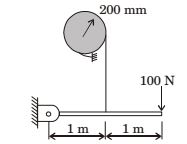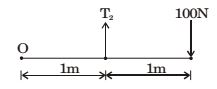## Machine Design Miscellaneous

#### Machine Design

Direction: A band brake consists of a lever attached to one end of the band. The other end of the band is fixed to the ground. The wheel has a radius of 200 mm and the wrap angle of the band is 270°. The braking force applied to the lever is limited to 100 N, and the coefficient of friction between the band and the wheel is 0.5. No other information is given.1. The maximum wheel torque that can be completely braked is

1. Braking torque = (T1 – T2) × r
= (2101 – 200) × 2 = 382 N–m

##### Correct Option: B

Braking torque = (T1 – T2) × r
= (2101 – 200) × 2 = 382 N–m

1. The maximum tension that can be generated in the band during braking is

1. θ = 270°
μ = 0.5∑ M0 = 0
⇒ T2 × 1 - 100 × 2 = 0
T2 = 200 N

 T1 = eμ θ T2

 T1 = e0.5 × (3π / 2) T2

T1 = 2110 N

##### Correct Option: B

θ = 270°
μ = 0.5∑ M0 = 0
⇒ T2 × 1 - 100 × 2 = 0
T2 = 200 N

 T1 = eμ θ T2

 T1 = e0.5 × (3π / 2) T2

T1 = 2110 N

1. In a band brake the ratio of tight side band tension to the tension on the slack side is 3. If the angle of overlap of band on the drum is 180°, the coefficient of friction required between drum and the band is

1.  Given , Tension in tight side = T1 = 3 Tension is slack side T2

 T1 = eμ θ T2

where, θ = angle of overlap = 180 = π radians
∴ 3 = eμ θ
ln(3) = μ θ
 ∴ μ = 1 ln(3) = 0.35 π

##### Correct Option: D

 Given , Tension in tight side = T1 = 3 Tension is slack side T2

 T1 = eμ θ T2

where, θ = angle of overlap = 180 = π radians
∴ 3 = eμ θ
ln(3) = μ θ
 ∴ μ = 1 ln(3) = 0.35 π

1. Which one of the following is used to convert a rotational motion into a translational motion?

1. NA

##### Correct Option: D

NA

1. For the given statements :
I. Mating spur gear teeth is an example of higher pair
II. A revolute Joint is an example of lower pair Indicate the correct answer.

1. Since higher pair has a line or point contact and lower pair has a surface of Area contact. Hence both are true. i.e., spur gear has line contact (Higher pair) and Revolute joint has surface contact (lower pair).

##### Correct Option: D

Since higher pair has a line or point contact and lower pair has a surface of Area contact. Hence both are true. i.e., spur gear has line contact (Higher pair) and Revolute joint has surface contact (lower pair).